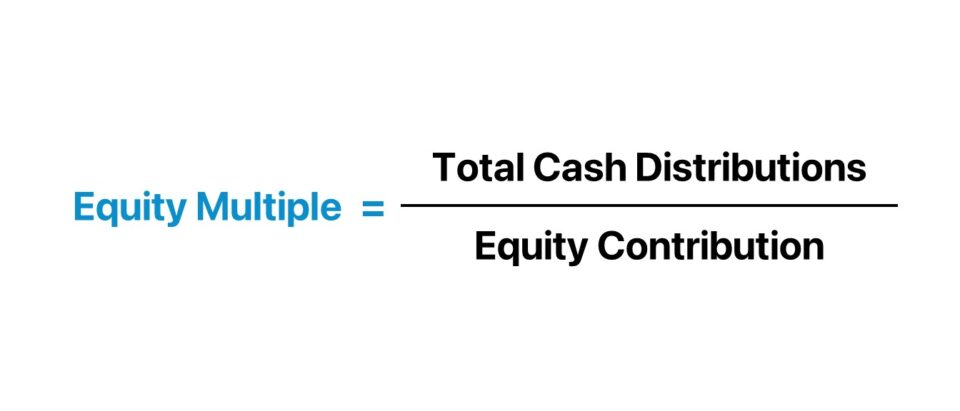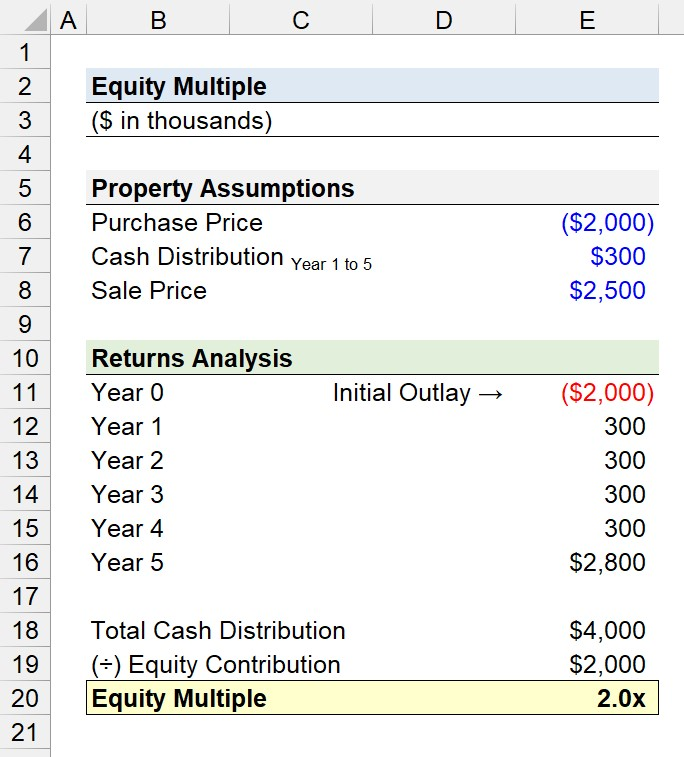Welcome to Wall Street Prep! Use code at checkout for 15% off.# Equity Multiple

Guide to Understanding the Equity Multiple## How to Calculate Equity Multiple?

The equity multiple is a real estate returns metric that compares the total cash distributions received by an investor on a property investment to the total capital contribution.

1. Total Cash Distributions (“Inflows”) → The cash retrieved from an investment over the holding period.
2. Equity Contribution (“Outflow”) → The total equity investment contributed by the investor on the original date of purchase.

In practice, the equity multiple is perceived to be a quick, “back of the envelope” method to analyze the return on a potential property investment.

The equity multiple is designed to compare the initial equity contribution of the investor to the total cash proceeds collected over the holding period.

The step-by-step process to calculate the equity multiple is as follows.

1. Determine the Total Equity Contribution (“Initial Outlay”)
2. Calculate the Sum of Cash Distributions Earned on the Investment
3. Divide the Total Cash Distributions by the Total Equity Contribution## Equity Multiple Formula

The equity multiple formula is straightforward, as it is the ratio between the total cash distributions and the total equity invested.

Equity Multiple = Total Cash Distributions ÷ Equity Contribution

Where:

• Total Cash Distribution → The cash “inflows” retrieved by the investor over the holding period of the property investment.
• Total Equity Contribution → The cash “outflows” incurred by the investor across the investment horizon of the property, namely the purchase price.

The equity multiple is expressed in absolute terms. Therefore, relying solely on the equity multiple to guide investment decisions can be costly.

## Equity Multiple vs. IRR: What is the Difference?

The notable drawback to the equity multiple is that the time value of money (or “TVM”), the core premise of the present value (PV) concept, is neglected in the ratio.

Thus, the equity multiple is insufficient to be a standalone return metric for analyzing investments, and must be used in conjunction with other metrics to truly understand the merits and risks of a given investment opportunity.

In particular, the internal rate of return (IRR) is complementary to the equity multiple to formulate a thesis on the profit potential of a potential property investment.

• Equity Multiple → The equity multiple is the ratio between the total return received from an investment and the initial investment. The ratio represents the number of times the initial investment is anticipated to be returned over the project (“turns”).
• Internal Rate of Return (IRR) → The IRR, on the other hand, is the annualized rate of return that would cause the net present value (NPV) of the project’s cash flows to be zero, i.e. the percentage by which an investment is expected to grow per annum. Unlike the equity multiple, the time value of money is factored into the IRR metric.

The IRR is therefore an annualized return that considers the time value of money (TVM), while the equity multiple is an easy-to-compute gross return figure. But the IRR and equity multiple each have their shortcomings, as seen in the following two scenarios:

1. High IRR + Low Equity Multiple → A property investment could yield a high IRR, but a sub-par equity multiple if the timing and cash proceeds were received earlier, i.e. the earlier receipt of cash distributions can distort the IRR metric.
2. Low IRR + High Equity Multiple → Conversely, a property investment could have a high equity multiple, but a lower IRR if the cash flows are spread across a longer time span.

## What is a Good Equity Multiple?

Conceptually, the equity multiple measures the earnings retrieved by an investor relative to the initial investment on a per-dollar basis.

For instance, an equity multiple of 2.5x implies that the investor should expect \$2.50 for each \$1.00 invested in a real estate project.

The general rules of thumb for interpreting the equity multiple are as follows.

General Guidelines Description
Equity Multiple = 1.0x
• If the equity multiple equals 1.0x, the investor is at the break-even point in terms of profitability (i.e. total cash distribution = total cash contribution).
• However, the opportunity cost of capital must be considered here, since the investor could have allocated the capital elsewhere to earn a higher return.
Equity Multiple < 1.0x
• If the equity multiple is sub-1.0x, that outcome would be viewed as unfavorable, because the investor received less cash than the initial investment amount, i.e. the investor has incurred a loss.
Equity Multiple > 1.0x
• If the equity multiple exceeds 1.0x, the investor has recovered their original investment in full, and any incremental cash distributions beyond the breakeven point are positive, i.e. “excess” returns.

## Equity Multiple vs. Cash on Cash Return: What is the Difference?

The cash-on-cash return and equity multiple are frequently misunderstood as interchangeable, but the two metrics serve different purposes and offer unique insights.

• Cash on Cash Return – The cash on cash return is the ratio between the annual pre-tax cash flow the investor earns on property investment and the invested equity in the coinciding period, expressed as a percentage.
• Equity Multiple – The equity multiple measures the total return on the investment and is calculated by dividing the total cash received by the total equity invested, i.e. the cash received per dollar invested.

The formula to calculate the cash on cash return is as follows.

Cash on Cash Return (CoC) = Annual Pre-Tax Cash Flow ÷ Invested Equity

The cash-on-cash return offers a “snapshot” of the annualized return relative to the cash investment, with consideration toward only the cash income generated by the property.

On the other hand, the equity multiple is the ratio between the total return – from the initial purchase date to the exit date – relative to the equity invested.

## Equity Multiple Calculator

We’ll now move on to a modeling exercise, which you can access by filling out the form below.#### Get the Excel Template!Submitting...

## Equity Multiple Calculation Example

Suppose a real estate investor acquired an existing building property for \$2 million.

From Year 1 to Year 5, the net cash proceeds attributable to the investor are fixed at \$300k each period.

• Property Purchase Price = \$2 million
• Cash Distribution (Year 1 to Year 5) = \$300k

If the property is sold at the end of Year 5 for \$2.5 million– a net increase of \$500k from the initial purchase price – what is the equity multiple?

The formula to compute the equity multiple requires only two inputs.

1. Total Cash Distribution
2. Equity Contribution

The total cash distribution is the sum of the positive cash flows from Years 1 to 5, which amounts to \$4 million.

• Property Sale Price = \$2.5 million
• Total Cash Distribution = (\$300k × 5) + \$2.5 million = \$4 million

The initial outlay in Year 0 – i.e. the purchase price of the property – should NOT be included in the calculation of the total cash distribution.

For Year 5, the property was sold at the end of the year, so the \$300k in annual cash distribution was included in the final year calculation.

In closing, we can divide the total cash distribution by the initial outlay to arrive at an implied equity multiple of 2.0x, which implies the equity contribution doubled in value over the investment holding period.

• Equity Multiple = \$4 million ÷ \$2 million = 2.0x20+ Hours of Online Video Training

### Master Real Estate Financial Modeling

This program breaks down everything you need to build and interpret real estate finance models. Used at the world's leading real estate private equity firms and academic institutions.

Inline Feedbacks